# Digital Logic - Combinational Circuits

51:

For the circuit shown for AB = 00,  AB = 01, C, S values respectively are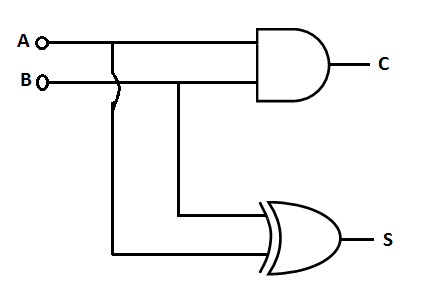A. 0 , 0 and 0, 1 B. 0, 0 and 1, 0 C. 0, 1 and 0, 0 D. 1, 0 and 0, 0 Answer Report Discuss Option: A Explanation : Click on Discuss to view users comments. Anilkumar said: (8:19am on Sunday 16th June 2013) for c A=0,B=0 the gate will be AND according AND property 0 0 =0 similarly in the case S the gate will be NOR gate if input is 1 1 the output are 1 similarly remain output 0 1 Write your comments here:
52:

What logic gate is represented by the circuit shown below?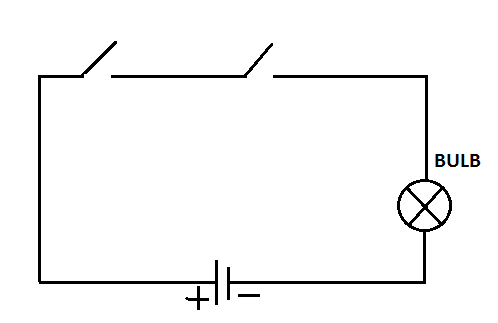A. NAND B. NOR C. AND D. EQUIVALENCE Answer Report Discuss Option: C Explanation : Click on Discuss to view users comments. vandna said: (10:30pm on Monday 9th September 2013) in AND gate output is 1 if and only if both inputs are 1.if we look at this circuit,it will allow to pass the current only if both switches are closed. aamir said: (2:31pm on Monday 31st October 2016) The 2 switches (say A and B) are connected in series, so if one of them or both is/are open the current won't flow via bulb . Write your comments here:
53:

The circuit shown below is the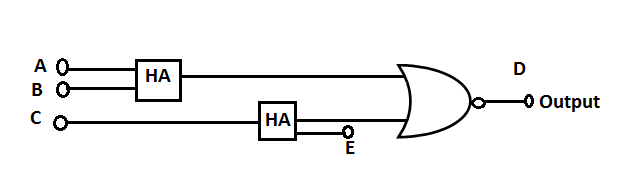A. Full adder B. Full subtractor C. Parity checker D. None of these Answer Report Discuss Option: A Explanation : Click on Discuss to view users comments. adit said: (2:06pm on Tuesday 14th May 2013) But it's truth table with A,B,C,E as inputs dont looks like full adder Write your comments here:
54:

In the  following gate network which gate is redundant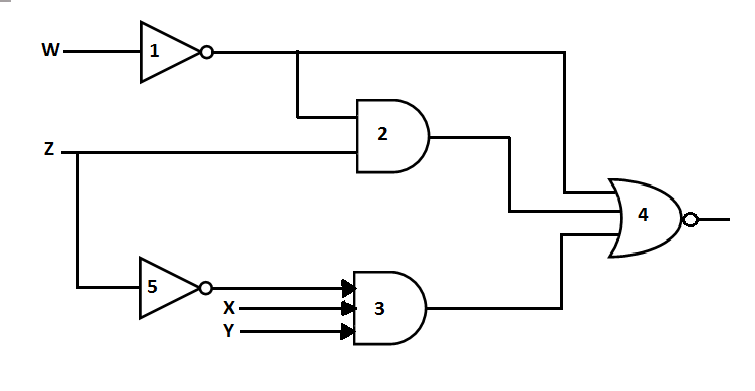A. Gate no. 1 B. Gate no. 2 C. Gate no. 3 D. Gate no. 4 Answer Report Discuss Option: B Explanation : Before Gate No. 4 being removed Output,   After Gate No. 4 being removed, Output,  Click on Discuss to view users comments. Write your comments here:
55:

The combinational circuit given below is implemented with two NAND gates. To which of the following individual gates is its equivalent?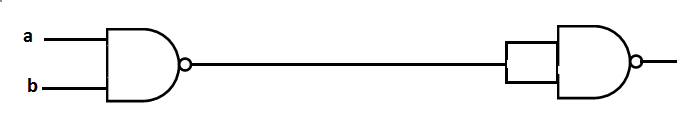A. NOT B. OR C. AND D. XOR Answer Report Discuss Option: C Explanation : [ (a.b) '. (a.b) ' ] '= ((a.b)')' + ((a.b)')' = ( a.b)+ (a.b) =(a.b) Click on Discuss to view users comments. Write your comments here: